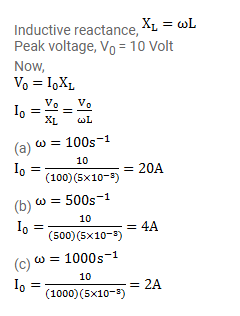# A coil of inductance 5.0mH and negligible resistance is connected

Question:

A coil of inductance $5.0 \mathrm{mH}$ and negligible resistance is connected to the oscillator of the previous problem. Find the peak currents in the circuit for $\omega=100 \mathrm{~s}^{-1}, 500 \mathrm{~s}^{-1}, 1000 \mathrm{~s}^{-1}$.

Solution: